# RD Sharma Solutions for Class 8 Maths Chapter 6 - Algebraic Expressions and Identities Exercise 6.2

RD Sharma Solutions for Class 8 Maths Exercise 6.2 of Chapter 6 Algebraic Expressions and Identities are available here in simple PDF. Students can download the RD Sharma Solutions pdf from the links provided below. BYJU’S expert team have solved and prepared the solutions in an easily understandable manner which help score good marks. In this Exercise 6.2 of Chapter 6 algebraic expressions and identities, we shall discuss the problems based on addition and subtraction of algebraic expressions.

## Download the pdf of RD Sharma Solutions For Class 8 Maths Exercise 6.2 Chapter 6 Algebraic Expressions and Identities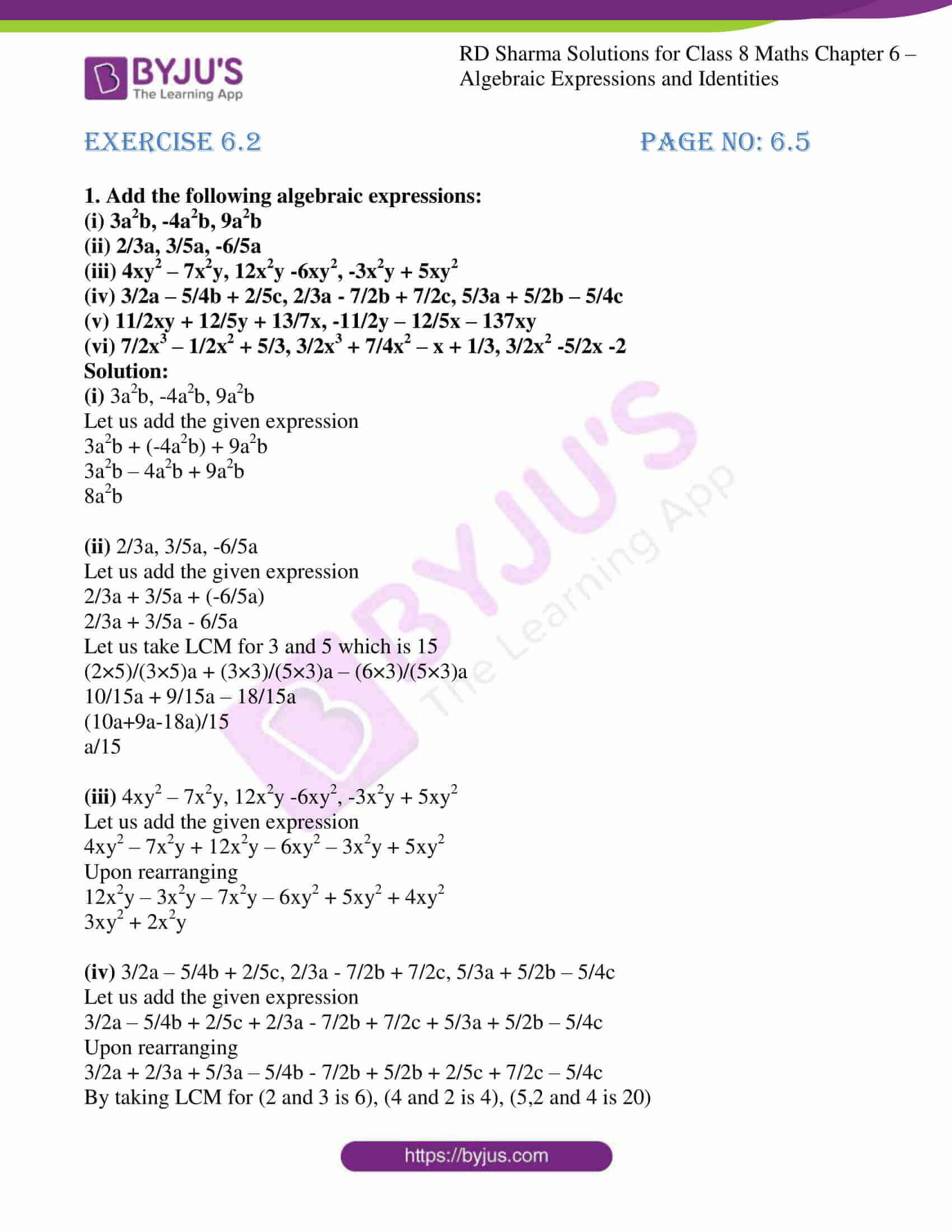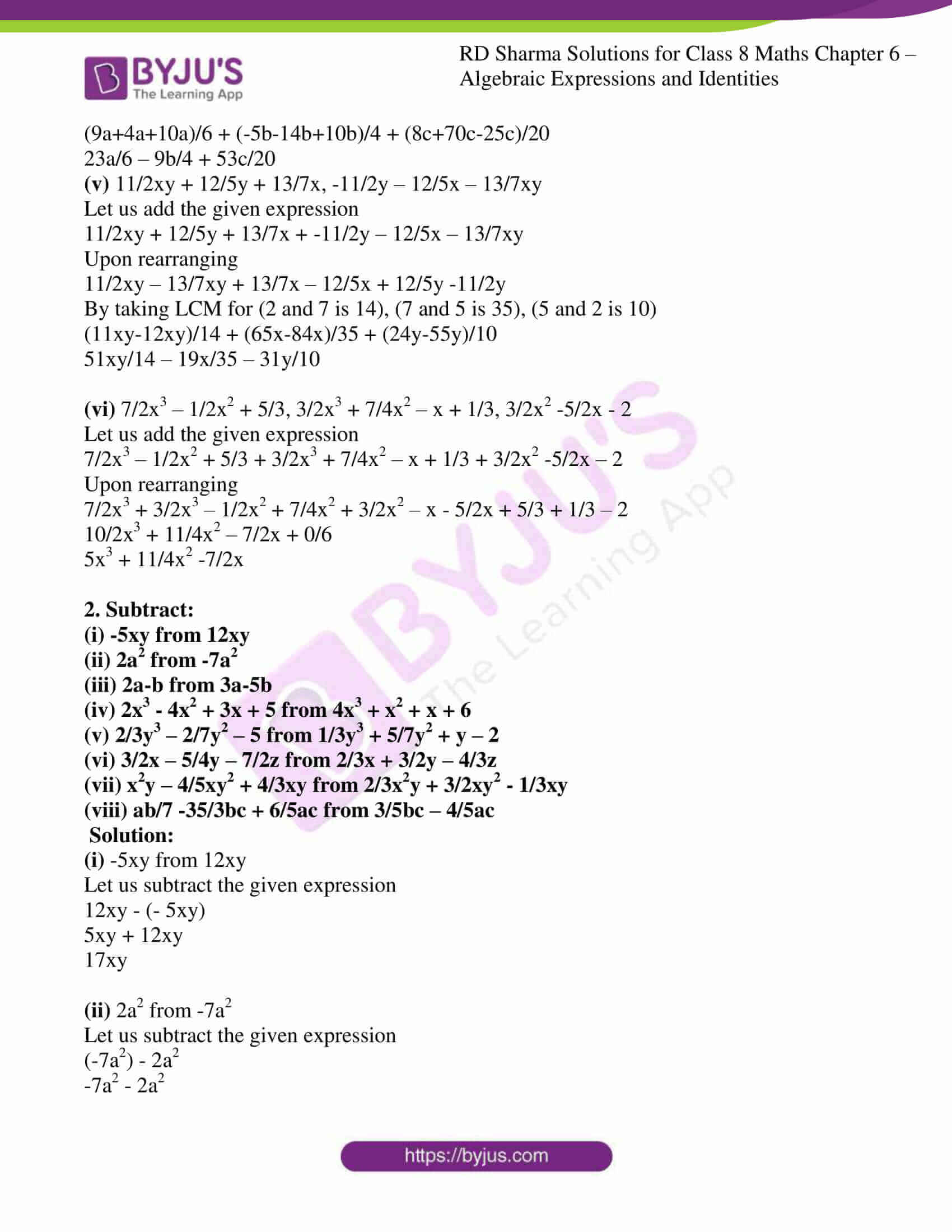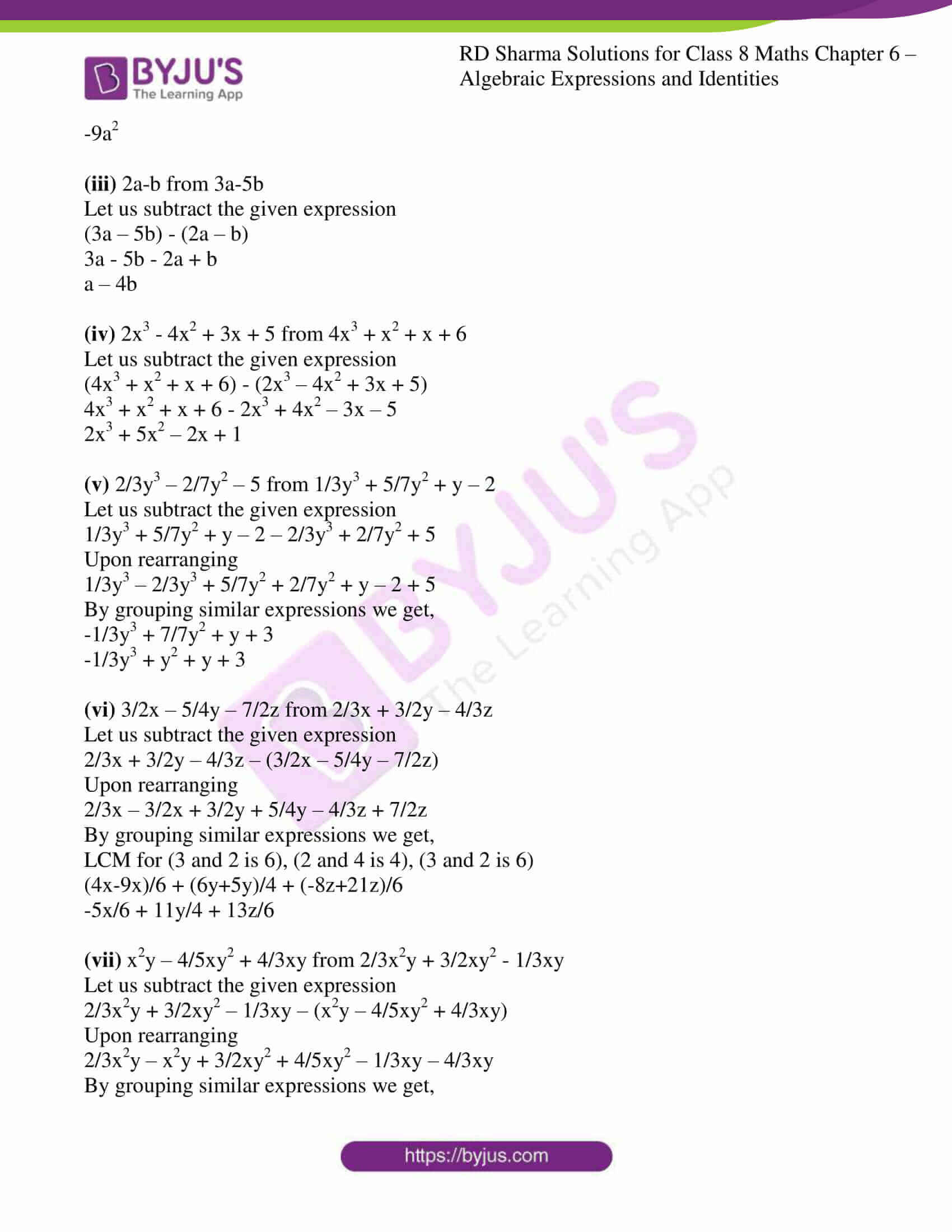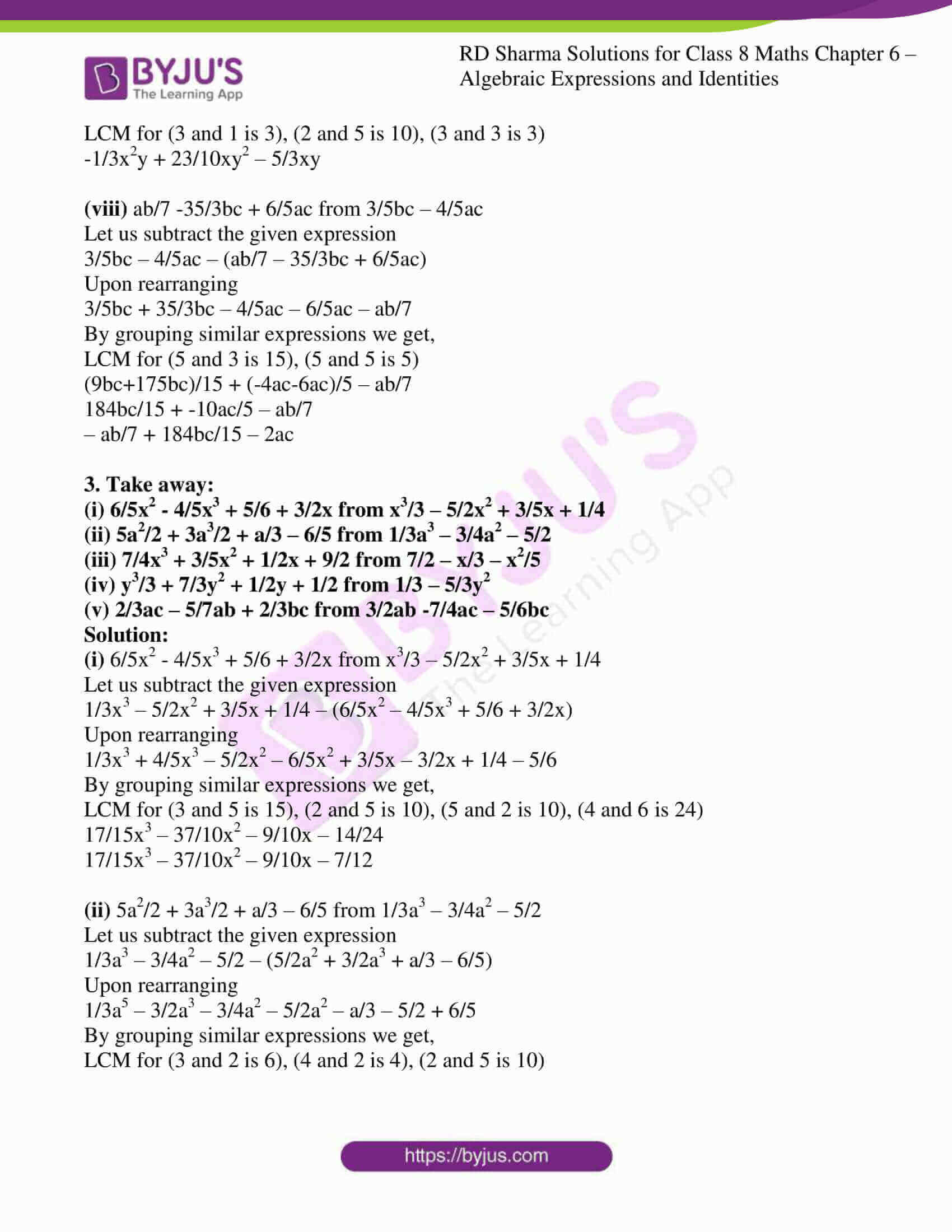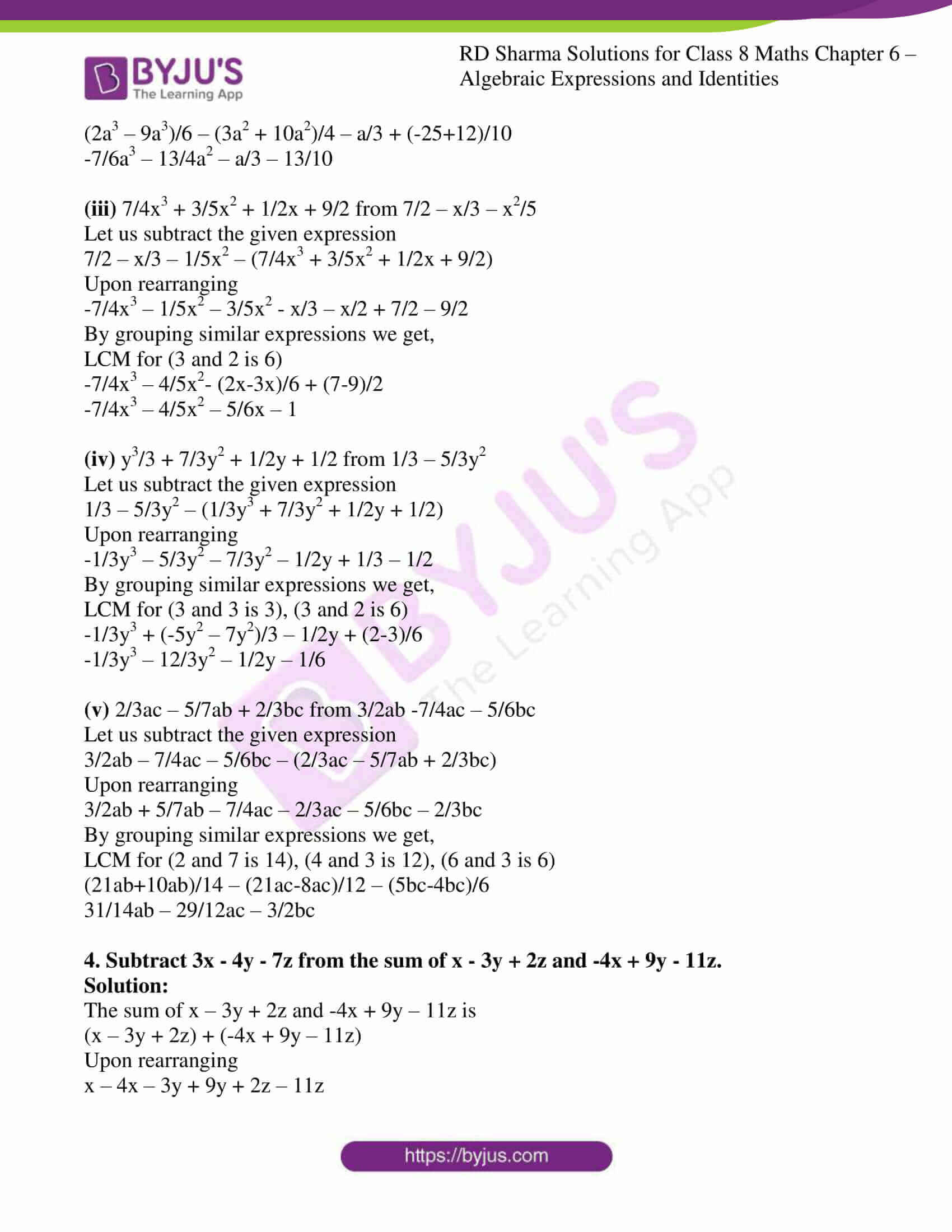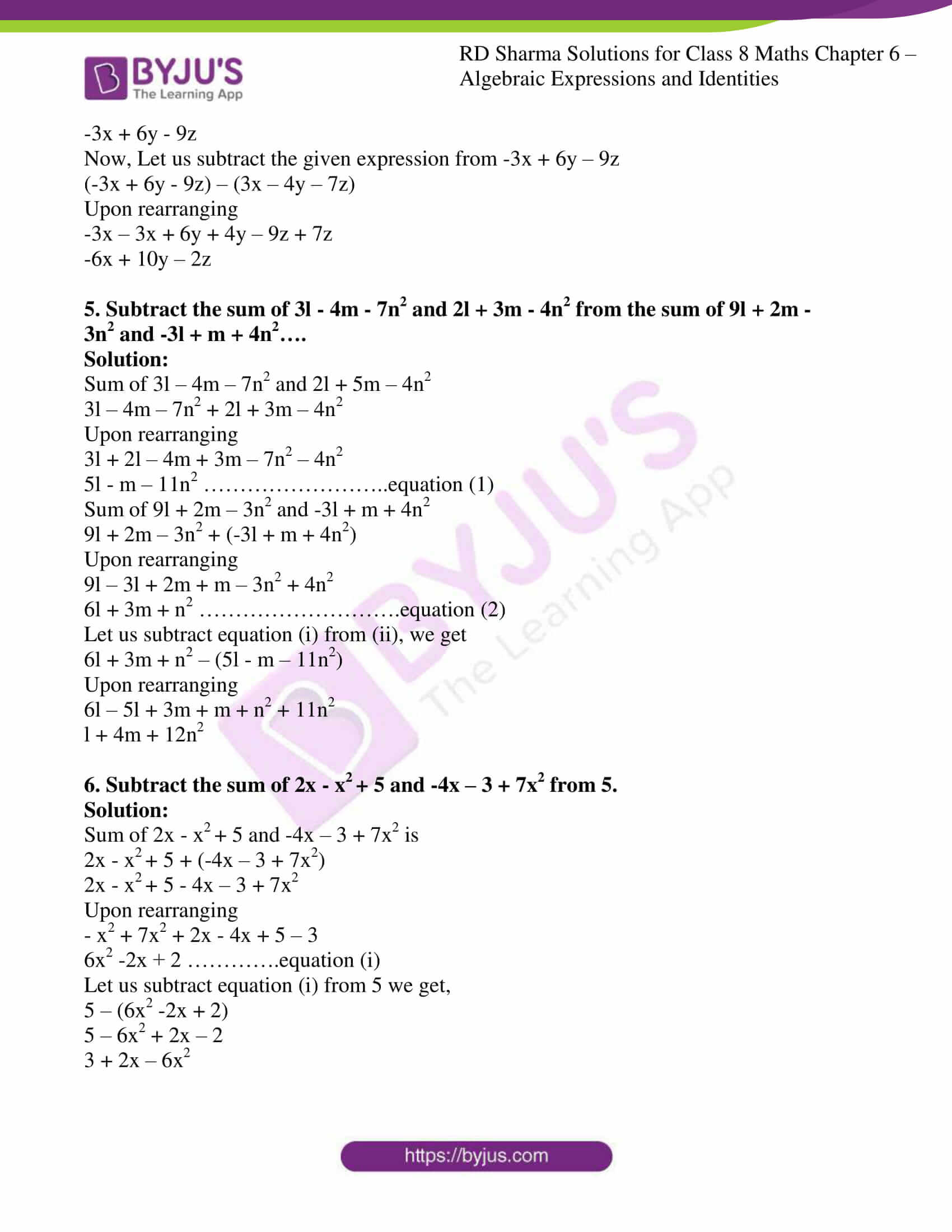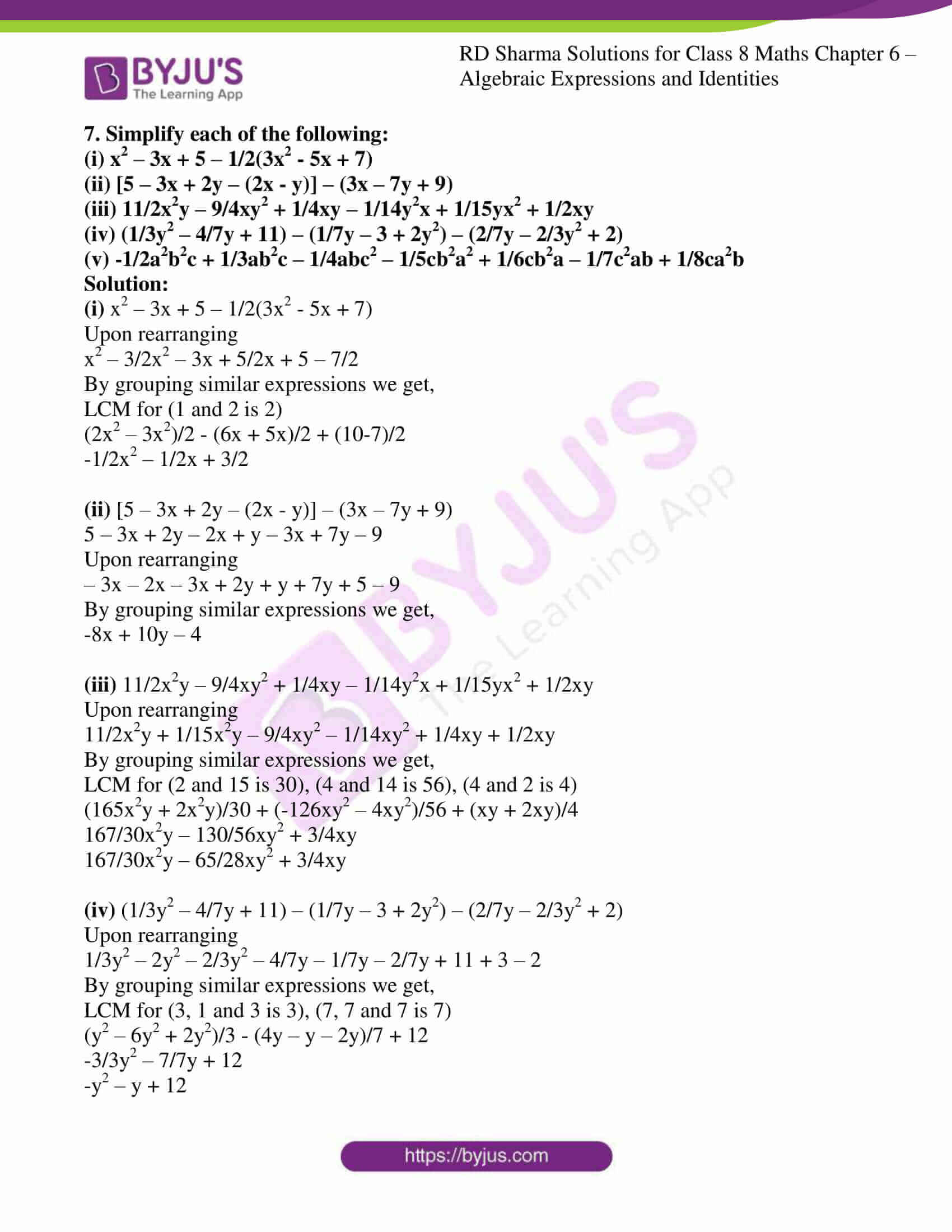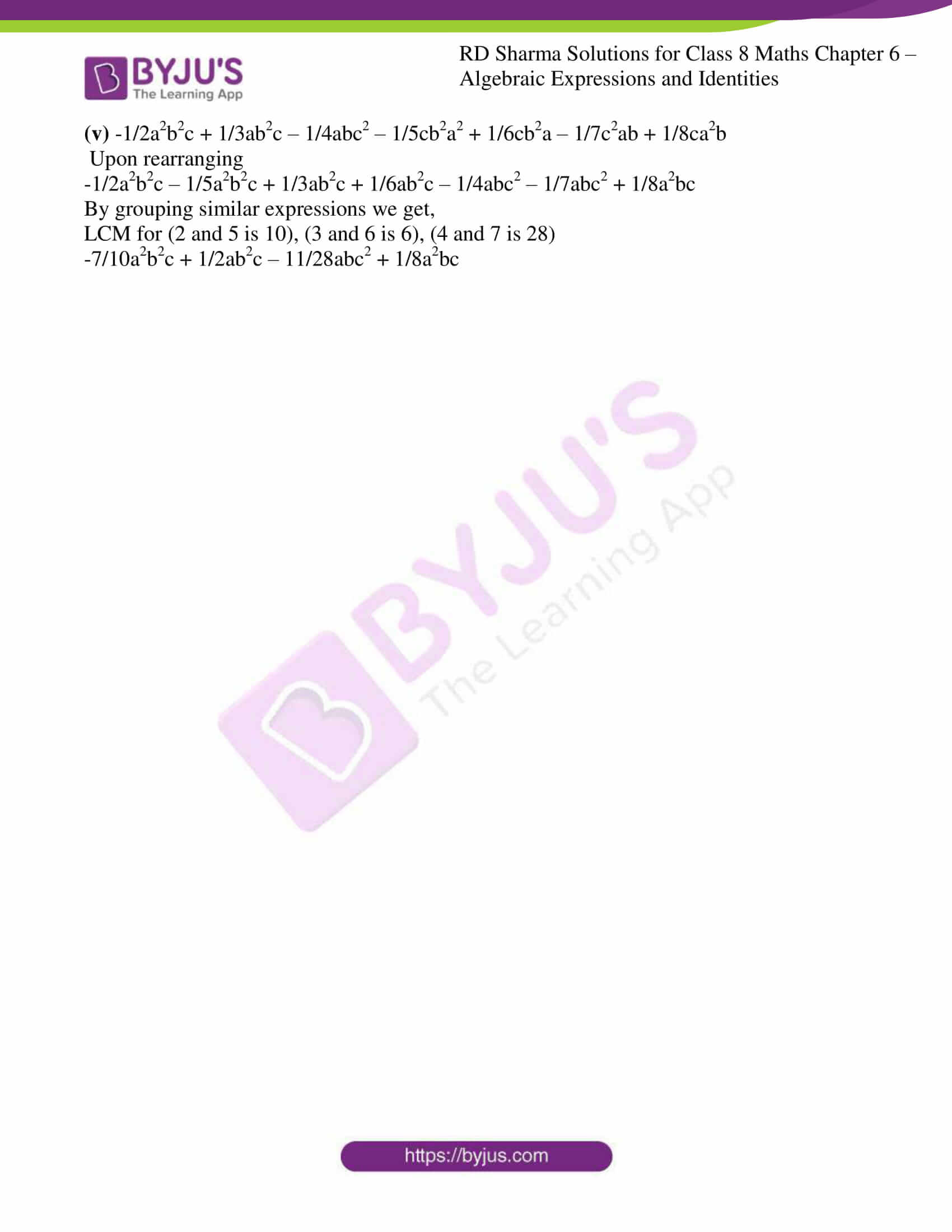### Access Answers to RD Sharma Solutions for Class 8 Maths Exercise 6.2 Chapter 6 Algebraic Expressions and Identities

#### EXERCISE 6.2 PAGE NO: 6.5

1. Add the following algebraic expressions:

(i) 3a2b, -4a2b, 9a2b

(ii) 2/3a, 3/5a, -6/5a

(iii) 4xy2 – 7x2y, 12x2y -6xy2, -3x2y + 5xy2

(iv) 3/2a – 5/4b + 2/5c, 2/3a – 7/2b + 7/2c, 5/3a + 5/2b – 5/4c

(v) 11/2xy + 12/5y + 13/7x, -11/2y – 12/5x – 137xy

(vi) 7/2x3 – 1/2x2 + 5/3, 3/2x3 + 7/4x2 – x + 1/3, 3/2x2 -5/2x -2

Solution:

(i) 3a2b, -4a2b, 9a2b

Let us add the given expression

3a2b + (-4a2b) + 9a2b

3a2b – 4a2b + 9a2b

8a2b

(ii) 2/3a, 3/5a, -6/5a

Let us add the given expression

2/3a + 3/5a + (-6/5a)

2/3a + 3/5a – 6/5a

Let us take LCM for 3 and 5 which is 15

(2×5)/(3×5)a + (3×3)/(5×3)a – (6×3)/(5×3)a

10/15a + 9/15a – 18/15a

(10a+9a-18a)/15

a/15

(iii) 4xy2 – 7x2y, 12x2y -6xy2, -3x2y + 5xy2

Let us add the given expression

4xy2 – 7x2y + 12x2y – 6xy2 – 3x2y + 5xy2

Upon rearranging

12x2y – 3x2y – 7x2y – 6xy2 + 5xy2 + 4xy2

3xy2 + 2x2y

(iv) 3/2a – 5/4b + 2/5c, 2/3a – 7/2b + 7/2c, 5/3a + 5/2b – 5/4c

Let us add the given expression

3/2a – 5/4b + 2/5c + 2/3a – 7/2b + 7/2c + 5/3a + 5/2b – 5/4c

Upon rearranging

3/2a + 2/3a + 5/3a – 5/4b – 7/2b + 5/2b + 2/5c + 7/2c – 5/4c

By taking LCM for (2 and 3 is 6), (4 and 2 is 4), (5,2 and 4 is 20)

(9a+4a+10a)/6 + (-5b-14b+10b)/4 + (8c+70c-25c)/20

23a/6 – 9b/4 + 53c/20

(v) 11/2xy + 12/5y + 13/7x, -11/2y – 12/5x – 13/7xy

Let us add the given expression

11/2xy + 12/5y + 13/7x + -11/2y – 12/5x – 13/7xy

Upon rearranging

11/2xy – 13/7xy + 13/7x – 12/5x + 12/5y -11/2y

By taking LCM for (2 and 7 is 14), (7 and 5 is 35), (5 and 2 is 10)

(11xy-12xy)/14 + (65x-84x)/35 + (24y-55y)/10

51xy/14 – 19x/35 – 31y/10

(vi) 7/2x3 – 1/2x2 + 5/3, 3/2x3 + 7/4x2 – x + 1/3, 3/2x2 -5/2x – 2

Let us add the given expression

7/2x3 – 1/2x2 + 5/3 + 3/2x3 + 7/4x2 – x + 1/3 + 3/2x2 -5/2x – 2

Upon rearranging

7/2x3 + 3/2x3 – 1/2x2 + 7/4x2 + 3/2x2 – x – 5/2x + 5/3 + 1/3 – 2

10/2x3 + 11/4x2 – 7/2x + 0/6

5x3 + 11/4x2 -7/2x

2. Subtract:
(i) -5xy from 12xy
(ii) 2a2 from -7a2
(iii) 2a-b from 3a-5b
(iv) 2x3 – 4x2 + 3x + 5 from 4x3 + x2 + x + 6
(v) 2/3y3 – 2/7y2 – 5 from 1/3y3 + 5/7y2 + y – 2
(vi) 3/2x – 5/4y – 7/2z from 2/3x + 3/2y – 4/3z
(vii) x2y – 4/5xy2 + 4/3xy from 2/3x2y + 3/2xy2 – 1/3xy
(viii) ab/7 -35/3bc + 6/5ac from 3/5bc – 4/5ac

Solution:

(i) -5xy from 12xy

Let us subtract the given expression

12xy – (- 5xy)

5xy + 12xy

17xy

(ii) 2a2 from -7a2

Let us subtract the given expression

(-7a2) – 2a2

-7a2 – 2a2

-9a2

(iii) 2a-b from 3a-5b

Let us subtract the given expression

(3a – 5b) – (2a – b)

3a – 5b – 2a + b

a – 4b

(iv) 2x3 – 4x2 + 3x + 5 from 4x3 + x2 + x + 6

Let us subtract the given expression

(4x3 + x2 + x + 6) – (2x3 – 4x2 + 3x + 5)

4x3 + x2 + x + 6 – 2x3 + 4x2 – 3x – 5

2x3 + 5x2 – 2x + 1

(v) 2/3y3 – 2/7y2 – 5 from 1/3y3 + 5/7y2 + y – 2

Let us subtract the given expression

1/3y3 + 5/7y2 + y – 2 – 2/3y3 + 2/7y2 + 5

Upon rearranging

1/3y3 – 2/3y3 + 5/7y2 + 2/7y2 + y – 2 + 5

By grouping similar expressions we get,

-1/3y3 + 7/7y2 + y + 3

-1/3y3 + y2 + y + 3

(vi) 3/2x – 5/4y – 7/2z from 2/3x + 3/2y – 4/3z

Let us subtract the given expression

2/3x + 3/2y – 4/3z – (3/2x – 5/4y – 7/2z)

Upon rearranging

2/3x – 3/2x + 3/2y + 5/4y – 4/3z + 7/2z

By grouping similar expressions we get,

LCM for (3 and 2 is 6), (2 and 4 is 4), (3 and 2 is 6)

(4x-9x)/6 + (6y+5y)/4 + (-8z+21z)/6

-5x/6 + 11y/4 + 13z/6

(vii) x2y – 4/5xy2 + 4/3xy from 2/3x2y + 3/2xy2 – 1/3xy

Let us subtract the given expression

2/3x2y + 3/2xy2 – 1/3xy – (x2y – 4/5xy2 + 4/3xy)

Upon rearranging

2/3x2y – x2y + 3/2xy2 + 4/5xy2 – 1/3xy – 4/3xy

By grouping similar expressions we get,

LCM for (3 and 1 is 3), (2 and 5 is 10), (3 and 3 is 3)

-1/3x2y + 23/10xy2 – 5/3xy

(viii) ab/7 -35/3bc + 6/5ac from 3/5bc – 4/5ac

Let us subtract the given expression

3/5bc – 4/5ac – (ab/7 – 35/3bc + 6/5ac)

Upon rearranging

3/5bc + 35/3bc – 4/5ac – 6/5ac – ab/7

By grouping similar expressions we get,

LCM for (5 and 3 is 15), (5 and 5 is 5)

(9bc+175bc)/15 + (-4ac-6ac)/5 – ab/7

184bc/15 + -10ac/5 – ab/7

– ab/7 + 184bc/15 – 2ac

3. Take away:

(i) 6/5x2 – 4/5x3 + 5/6 + 3/2x from x3/3 – 5/2x2 + 3/5x + 1/4

(ii) 5a2/2 + 3a3/2 + a/3 – 6/5 from 1/3a3 – 3/4a2 – 5/2

(iii) 7/4x3 + 3/5x2 + 1/2x + 9/2 from 7/2 – x/3 – x2/5
(iv) y3/3 + 7/3y2 + 1/2y + 1/2 from 1/3 – 5/3y2

(v) 2/3ac – 5/7ab + 2/3bc from 3/2ab -7/4ac – 5/6bc

Solution:

(i) 6/5x2 – 4/5x3 + 5/6 + 3/2x from x3/3 – 5/2x2 + 3/5x + 1/4

Let us subtract the given expression

1/3x3 – 5/2x2 + 3/5x + 1/4 – (6/5x2 – 4/5x3 + 5/6 + 3/2x)

Upon rearranging

1/3x3 + 4/5x3 – 5/2x2 – 6/5x2 + 3/5x – 3/2x + 1/4 – 5/6

By grouping similar expressions we get,

LCM for (3 and 5 is 15), (2 and 5 is 10), (5 and 2 is 10), (4 and 6 is 24)

17/15x3 – 37/10x2 – 9/10x – 14/24

17/15x3 – 37/10x2 – 9/10x – 7/12

(ii) 5a2/2 + 3a3/2 + a/3 – 6/5 from 1/3a3 – 3/4a2 – 5/2

Let us subtract the given expression

1/3a3 – 3/4a2 – 5/2 – (5/2a2 + 3/2a3 + a/3 – 6/5)

Upon rearranging

1/3a5 – 3/2a3 – 3/4a2 – 5/2a2 – a/3 – 5/2 + 6/5

By grouping similar expressions we get,

LCM for (3 and 2 is 6), (4 and 2 is 4), (2 and 5 is 10)

(2a3 – 9a3)/6 – (3a2 + 10a2)/4 – a/3 + (-25+12)/10

-7/6a3 – 13/4a2 – a/3 – 13/10

(iii) 7/4x3 + 3/5x2 + 1/2x + 9/2 from 7/2 – x/3 – x2/5

Let us subtract the given expression

7/2 – x/3 – 1/5x2 – (7/4x3 + 3/5x2 + 1/2x + 9/2)

Upon rearranging

-7/4x3 – 1/5x2 – 3/5x2 – x/3 – x/2 + 7/2 – 9/2

By grouping similar expressions we get,

LCM for (3 and 2 is 6)

-7/4x3 – 4/5x2– (2x-3x)/6 + (7-9)/2

-7/4x3 – 4/5x2 – 5/6x – 1

(iv) y3/3 + 7/3y2 + 1/2y + 1/2 from 1/3 – 5/3y2

Let us subtract the given expression

1/3 – 5/3y2 – (1/3y3 + 7/3y2 + 1/2y + 1/2)

Upon rearranging

-1/3y3 – 5/3y2 – 7/3y2 – 1/2y + 1/3 – 1/2

By grouping similar expressions we get,

LCM for (3 and 3 is 3), (3 and 2 is 6)

-1/3y3 + (-5y2 – 7y2)/3 – 1/2y + (2-3)/6

-1/3y3 – 12/3y2 – 1/2y – 1/6

(v) 2/3ac – 5/7ab + 2/3bc from 3/2ab -7/4ac – 5/6bc

Let us subtract the given expression

3/2ab – 7/4ac – 5/6bc – (2/3ac – 5/7ab + 2/3bc)

Upon rearranging

3/2ab + 5/7ab – 7/4ac – 2/3ac – 5/6bc – 2/3bc

By grouping similar expressions we get,

LCM for (2 and 7 is 14), (4 and 3 is 12), (6 and 3 is 6)

(21ab+10ab)/14 – (21ac-8ac)/12 – (5bc-4bc)/6

31/14ab – 29/12ac – 3/2bc

4. Subtract 3x – 4y – 7z from the sum of x – 3y + 2z and -4x + 9y – 11z.

Solution:

The sum of x – 3y + 2z and -4x + 9y – 11z is

(x – 3y + 2z) + (-4x + 9y – 11z)

Upon rearranging

x – 4x – 3y + 9y + 2z – 11z

-3x + 6y – 9z

Now, Let us subtract the given expression from -3x + 6y – 9z

(-3x + 6y – 9z) – (3x – 4y – 7z)

Upon rearranging

-3x – 3x + 6y + 4y – 9z + 7z

-6x + 10y – 2z

5. Subtract the sum of 3l – 4m – 7n2 and 2l + 3m – 4n2 from the sum of 9l + 2m – 3n2 and -3l + m + 4n2….

Solution:

Sum of 3l – 4m – 7n2 and 2l + 5m – 4n2

3l – 4m – 7n2 + 2l + 3m – 4n2

Upon rearranging

3l + 2l – 4m + 3m – 7n2 – 4n2

5l – m – 11n2 ……………………..equation (1)

Sum of 9l + 2m – 3n2 and -3l + m + 4n2

9l + 2m – 3n2 + (-3l + m + 4n2)

Upon rearranging

9l – 3l + 2m + m – 3n2 + 4n2

6l + 3m + n2 ……………………….equation (2)

Let us subtract equation (i) from (ii), we get

6l + 3m + n2 – (5l – m – 11n2)

Upon rearranging

6l – 5l + 3m + m + n2 + 11n2

l + 4m + 12n2

6. Subtract the sum of 2x – x2 + 5 and -4x – 3 + 7x2 from 5.

Solution:

Sum of 2x – x2 + 5 and -4x – 3 + 7x2 is

2x – x2 + 5 + (-4x – 3 + 7x2)

2x – x2 + 5 – 4x – 3 + 7x2

Upon rearranging

– x2 + 7x2 + 2x – 4x + 5 – 3

6x2 -2x + 2 ………….equation (i)

Let us subtract equation (i) from 5 we get,

5 – (6x2 -2x + 2)

5 – 6x2 + 2x – 2

3 + 2x – 6x2

7. Simplify each of the following:

(i) x2 – 3x + 5 – 1/2(3x2 – 5x + 7)

(ii) [5 – 3x + 2y – (2x – y)] – (3x – 7y + 9)

(iii) 11/2x2y – 9/4xy2 + 1/4xy – 1/14y2x + 1/15yx2 + 1/2xy

(iv) (1/3y2 – 4/7y + 11) – (1/7y – 3 + 2y2) – (2/7y – 2/3y2 + 2)

(v) -1/2a2b2c + 1/3ab2c – 1/4abc2 – 1/5cb2a2 + 1/6cb2a – 1/7c2ab + 1/8ca2b

Solution:

(i) x2 – 3x + 5 – 1/2(3x2 – 5x + 7)

Upon rearranging

x2 – 3/2x2 – 3x + 5/2x + 5 – 7/2

By grouping similar expressions we get,

LCM for (1 and 2 is 2)

(2x2 – 3x2)/2 – (6x + 5x)/2 + (10-7)/2

-1/2x2 – 1/2x + 3/2

(ii) [5 – 3x + 2y – (2x – y)] – (3x – 7y + 9)

5 – 3x + 2y – 2x + y – 3x + 7y – 9

Upon rearranging

– 3x – 2x – 3x + 2y + y + 7y + 5 – 9

By grouping similar expressions we get,

-8x + 10y – 4

(iii) 11/2x2y – 9/4xy2 + 1/4xy – 1/14y2x + 1/15yx2 + 1/2xy

Upon rearranging

11/2x2y + 1/15x2y – 9/4xy2 – 1/14xy2 + 1/4xy + 1/2xy

By grouping similar expressions we get,

LCM for (2 and 15 is 30), (4 and 14 is 56), (4 and 2 is 4)

(165x2y + 2x2y)/30 + (-126xy2 – 4xy2)/56 + (xy + 2xy)/4

167/30x2y – 130/56xy2 + 3/4xy

167/30x2y – 65/28xy2 + 3/4xy

(iv) (1/3y2 – 4/7y + 11) – (1/7y – 3 + 2y2) – (2/7y – 2/3y2 + 2)

Upon rearranging

1/3y2 – 2y2 – 2/3y2 – 4/7y – 1/7y – 2/7y + 11 + 3 – 2

By grouping similar expressions we get,

LCM for (3, 1 and 3 is 3), (7, 7 and 7 is 7)

(y2 – 6y2 + 2y2)/3 – (4y – y – 2y)/7 + 12

-3/3y2 – 7/7y + 12

-y2 – y + 12

(v) -1/2a2b2c + 1/3ab2c – 1/4abc2 – 1/5cb2a2 + 1/6cb2a – 1/7c2ab + 1/8ca2b

Upon rearranging

-1/2a2b2c – 1/5a2b2c + 1/3ab2c + 1/6ab2c – 1/4abc2 – 1/7abc2 + 1/8a2bc

By grouping similar expressions we get,

LCM for (2 and 5 is 10), (3 and 6 is 6), (4 and 7 is 28)

-7/10a2b2c + 1/2ab2c – 11/28abc2 + 1/8a2bc